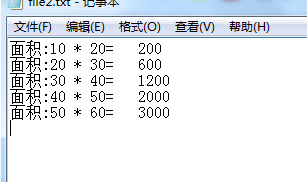• ## python计算矩形面积

万次阅读 2019-12-03 11:58:08
class Rectangle(object): def __init__(self,width=0,height=0): self.width=width self.height=height def __setattr__(self,name,value): if name=='square': self...
class Rectangle(object):
def __init__(self,width=0,height=0):
self.width=width
self.height=height

def __setattr__(self,name,value):
if name=='square':
self.width=value
else:
self.__dict__[name]=value
##          super(Rectangle,self).__setattr__(name,value)
def getArea(self):
return self.width*self.height


展开全文• 利用 python 计算矩形面积的交并比，利用 python 简单计算矩形面积的交并比，
• 今天小编就为大家分享一篇python实现用类读取文件数据并计算矩形面积，具有很好的参考价值，希望对大家有所帮助。一起跟随小编过来看看吧
• 这篇文章主要介绍了Python 实现两个矩形重合面积代码实例,文中通过示例代码介绍的非常详细，对大家的学习或者工作具有一定的参考学习价值,需要的朋友可以参考下 代码如下 计算两个矩形的重合面积 import math x1, ...
• Python】第1次作业：圆面积的计算A，计算矩形面积，说句心里话A 1、圆面积的计算A 题目：根据圆半径计算圆面积，结果保留两位小数。其中，圆周率使用 3.1415。 raduis=float(input()) area=3.1415*raduis*raduis ...
【Python】第1次作业：圆面积的计算A，计算矩形面积，说句心里话A
1、圆面积的计算A
题目：根据圆半径计算圆面积，结果保留两位小数。其中，圆周率使用 3.1415。
raduis=float(input())
area=3.1415*raduis*raduis
print(format(area,'.2f'))
2、计算矩形面积
题目：用户输入矩形的长和宽，计算其面积并输出，结果四舍五入，保留2位小数。
a=float(input())
b=float(input())
s=a*b
print(format(s,'.2f'))
3、说句心里话A
题目：分两次从控制台接收用户的两个输入：第一个内容为"人名"，第二个内容为"心里话"。‪‬‪‬‪‬‪‬‪‬‮‬‪‬‫‬‪‬‪‬‪‬‪‬‪‬‮‬‪‬‫‬‪‬‪‬‪‬‪‬‪‬‮‬‫‬‫‬‪‬‪‬‪‬‪‬‪‬‮‬‭‬‫‬‪‬‪‬‪‬‪‬‪‬‮‬‪‬‫‬‪‬‪‬‪‬‪‬‪‬‮‬‪‬‮‬然后将这两个输入内容组成如下句型并输出出来：‪‬‪‬‪‬‪‬‪‬‮‬‪‬‫‬‪‬‪‬‪‬‪‬‪‬‮‬‪‬‫‬‪‬‪‬‪‬‪‬‪‬‮‬‫‬‫‬‪‬‪‬‪‬‪‬‪‬‮‬‭‬‫‬‪‬‪‬‪‬‪‬‪‬‮‬‪‬‫‬‪‬‪‬‪‬‪‬‪‬‮‬‪‬‮‬      (人名)，我想对你说，(心里话)
name=input()
heartword=input()
str1=name+'，我想对你说，'+heartword
print(str1)


展开全文• 1.创建一个类Rectangle，已知a、b求面积，求三角形的面积 ...'''这是关于矩形面积计算公式，只用给出矩形的长和宽， 调用实例函数，就可以返回所需要的面积’’’ number=0 def __init__(self...
1.创建一个类Rectangle，已知a、b求面积，求三角形的面积
2.结合题目一，从题目一文件中读取数据，并采用类的方法，将计算的结果写在另一个文档中。
(1)利用类进行计算一个矩形的面积，已经a、b边长。
class Rectangle:
'''这是关于矩形面积的计算公式，只用给出矩形的长和宽，

调用实例函数，就可以返回所需要的面积’’’
number=0
def __init__(self,a,b):  # a、b类似C中的形参  或者叫作构造方法
self.a1=a            # 将形参中的值传入到类模板
self.b1=b            # 实例变量
Rectangle.number=Rectangle.number+1
self.no=Rectangle.number

def getArear(self):
s1=self.a1
s2=self.b1
area=s1*s2
return area

r1=Rectangle(10,20)
print(r1.a1)
s=r1.getArear()
print(s)
print(Rectangle.doc)
print(r1.number)
执行结果：
（2）结合第一题，从文件中读取数据进行计算
class Rectangle:
'''这是关于矩形面积的计算公式，只用给出矩形的长和宽，

调用实例函数，就可以返回所需要的面积’’’
number=0
def __init__(self,a,b):  # a、b类似C中的形参  或者叫作构造方法
self.a1=a            # 将形参中的值传入到类模板
self.b1=b            # 实例变量

def getArear(self):
s1=self.a1
s2=self.b1
area=s1*s2
return area

print(Rectangle.doc)
print(’\n’)
file1=open(’./Test2/file1.txt’)
str1=file1.readlines()
file2=open(’./Test2/file2.txt’,‘w’)          # 以文件覆盖的形式进行写入
for line in str1:
x=line.split()
r1=Rectangle(int(x),int(x))  # 直接调用类定义函数
area=r1.getArear()
print(area)                        # 调用类变量函数
file2.write(‘面积:%d * %d=\t’%(int(x),int(x))) # 所在行
file2.write(str(area))
file2.write(str(area))
file2.write('\n')

file2.close()
执行结果如下：
这是关于矩形面积的计算公式，只用给出矩形的长和宽，
调用实例函数，就可以返回所需要的面积
200
600
1200
2000
3000展开全文• 题目：原题链接（简单） ...LeetCode的Python执行用时随缘，只要时间复杂度没有明显差异，执行用时一般都在同一个量级，仅作参考意义。 解法一（暴力解法）： def constructRectangle(self, area: int)
题目：原题链接（简单）
题目标签：

解法
时间复杂度
空间复杂度
执行用时

Ans 1 (Python)
O(n)
O(1)
2488ms (10.92%)

Ans 2 (Python)
$O(\sqrt{n})$
O(1)
36ms (93.80%)

LeetCode的Python执行用时随缘，只要时间复杂度没有明显差异，执行用时一般都在同一个量级，仅作参考意义。

解法一（暴力解法）：
def constructRectangle(self, area: int) -> List[int]:
c = pow(area, 0.5)
l = w = int(c)  # l>w
while l * w != area:
if l * w < area:
l += 1
else:
w -= 1
return [l, w]

解法二（遍历解法）：
def constructRectangle(self, area: int) -> List[int]:
c = int(pow(area, 0.5))
while area % c != 0:
c -= 1
else:
return [c, area // c]



展开全文leetcode 算法
• 计算交并比：交的面积除以并的面积。 要求矩形框的长和宽应该平行于图片框。不然不能用这样的公式计算。 原理，从一维上来理解：两条红线的距离之和减去黑色线之间的距离就是相交的距离。两条红线之和很容易算，两条...
• 计算交并比：交的面积除以并的面积。 要求矩形框的长和宽应该平行于图片框。不然不能用这样的公式计算。 原理，从一维上来理解：两条红线的距离之和减去黑色线之间的距离就是相交的距离。两条红线之和很容易算，两...inter 目标检测
• 这篇文章主要介绍了Python计算不规则图形面积算法实现解析,文中通过示例代码介绍的非常详细，对大家的学习或者工作具有一定的参考学习价值,需要的朋友可以参考下 介绍：大三上做一个医学影像识别的项目，医生在原图...
• 在图像处理中，经常需要计算两个矩形的重叠面积，在 python 中，可以使用 shapely 包中的 Polygon 函数，但是到了 c++ 没有想象中的那么简单。 查阅了很多资料，基本上都是判断两个矩形是否包含来计算，但是两个矩形...
• 在二维平面上计算出两个由直线构成的矩形重叠后形成的总面积。...说明: 假设矩形面积不会超出int的范围。 来源：力扣（LeetCode） 链接：https://leetcode-cn.com/problems/rectangle-area 著作权归领扣网络所...数学
• 在二维平面上计算出两个由直线构成的矩形重叠后形成的总面积。 每个矩形由其左下顶点和右上顶点坐标表示，如图所示。 示例: 输入: -3, 0, 3, 4, 0, -1, 9, 2 输出: 45 思考 法一：如何两个矩形相交，则计算相交后的...leetcode 算法
• 223. 矩形面积 在二维平面上计算出两个由直线构成的矩形重叠后形成的总面积。 每个矩形由其左下顶点和右上顶点坐标表示，如图所示。 示例: 输入: -3, 0, 3, 4, 0, -1, 9, 2 输出: 45 说明: 假设矩形面积...leetcode
• # 计算圆的面积 def circle_area(radius): return math.pi * radius * radius # 计算圆的周长 def circle_perimeter(radius): return math.pi * radius * 2 rad = float(input("请输入圆的半径： ")) if rad <...
• 计算两个矩形的重合面积 import math x1, y1, x2, y2 = input().split(" ") x1, y1, x2, y2=int(x1), int(y1), int(x2), int(y2) # print(x1, y1, x2, y2) x1,x2 = min(x1,x2),max(x1,x2) y1,y2= min(y1,y2)...
• python计算iou以及nms iou iou即交并比，如下图所示： 就是拿两个矩形的交集/并集，我们设交集为inner_area,矩形1面积为area1,矩形1面积为area2,则对应iou为inner_area/(area1+area2-inner_area) ,而两个矩形面积...numpy 目标检测
• Python几何形状区域 这是一个使用Python为学校作业分配的程序。该项目的目的是开发代码，以使用用户给定的输入来计算矩形，三角形和圆形的面积
• cv2.contourArea(cnt， True) # 计算轮廓的面积 参数说明：cnt为输入的单个轮廓值 cv2.arcLength(cnt， True) # 计算轮廓的周长 参数说明：cnt为输入的单个轮廓值 cv2.aprroxPolyDP(cnt, epsilon， True) # 用于获得...opencv
• 首先构造一个单位正方形和一个四分之一圆，然后随机想其中抛洒大量的点，每个点可能在圆内也可能在圆外，当点数足够多时圆内点将构成圆的面积，全部点将构成矩形面积，用圆内点数量除以全部点数量，就得到了π/4。...
• 5. 将点集中的点进行三角化，可排成逆时针，计算面积； 6. 此时的IOU为。 使用Python中的Shapely模块可轻松地进行Skew IOU Computation： from shapely.geometry import Polygon def intersection(g, p):
• 请你帮助牛牛计算下最小矩形面积。 输入描述: 首先输入一个正整数N表示点的个数（2 <= N <= 50） 接下来N行每行两个整数x, y，表示该点的坐标。绝对值均小于等于100. 输出描述: 一个整数表示最小矩形面积...
• Python程序，用于计算三角形，正方形和矩形＃2的面积。 解决二次方程式＃3的Python程序。 交换两个变量的Python程序＃4。 生成随机数＃5的Python程序。 Python程序，可将公里转换为英里，将摄氏度转换为华氏度，...# python计算矩形面积python 订阅